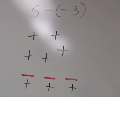This is a series of lessons fouced on

• Making sense of integer subtraction using number lines, patterns, and the chip model

It contains two activities and homework

• Part 1 - Number lines and Patterns
• Part 2 - Chip Model
• Homework

To see a related video, go to:

http://vimeo.com/71450580

Subtracting Integers

Created on 9/23/2014 by Scott Adamson

This is a series of lessons fouced on

• Making sense of integer subtraction using number lines, patterns, and the chip model

It contains two activities and homework

• Part 1 - Number lines and Patterns
• Part 2 - Chip Model
• Homework

To see a related video, go to:

http://vimeo.com/71450580